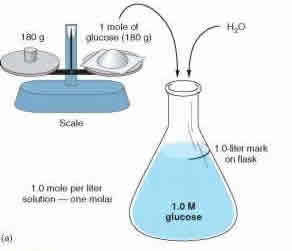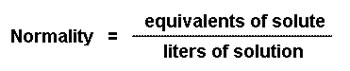# Difference between Molarity and Normality

##### Key Difference: Molarity, also known as molar concentration, measures the number of moles of a substance present in per liter of solution. Molarity is denoted with a capital M. Normality is basically a measure of concentration that is equal to the gram equivalent weight per liter of solution.Morality and normality are two methods of measuring concentration. These two concepts play an important part in science, specifically chemistry and help us break down mixtures and solutions. These two measurements help us determine how much of which substance is dissolved in another substance.

Both of the concepts base their calculations on how many moles are present in the current solution. A solution can be defined as a homogeneous mixture, which means two or more components have been mixed to the point that it cannot be distinguished by the naked eye. These two concepts require understanding moles as it determines the number of moles present in the solution. The theory of moles was discovered by Italian scientist, Amedeo Avogardo.

In 1811, Avogardo proposed that the volume of gas, at a given temperature and pressure, is proportional to the number of atoms or molecules regardless of its nature. This concept is known as the Avogardo constant. It is defined as the number of constituent particles (usually atoms or molecules) in one mole of a given substance. In laymen terms, one mole of a substance would represent the number of atoms and molecules present in the element. For example: Oxygen has an atomic weight of 16, this would represent the mass of per mole of oxygen. So, one mole of oxygen would have a mass of 16 grams. According to Avogardo, one mole of oxygen would have the same number of atoms as one mole of hydrogen. However, they can differ in weight.

Molarity and normality measures the concentration using two different approaches. Molarity, also known as molar concentration, measures the number of moles of a substance present in per liter of solution. Molarity is denoted with a capital M. So a solution with 1.0M would state that it contains 1 mole of solute per liter of solution. For example: A solution of water and sugar. If the solution is measured as 1.0 M, then the solution has 1 mole of sugar per liter of solution present. Molarity is also known as amount-of-substance concentration, amount concentration, substance concentration or simply concentration. Molarity can be calculated using the formula: M = mol (# of moles) / L (volume of solution in liters).

Normality is basically a measure of concentration that is equal to the gram equivalent weight per liter of solution. An equivalent is the number of moles of reactive units in a compound. Gram equivalent weight is a measure of the reactive capacity of a molecule. According to chemeketa.edu, “The normality of a solution is the concentration expressed as the number of equivalent weights (equivalents) of solute per liter of solution.” Normality is denoted with a capital N. In laymen’s terms, normality of an acid is how many H+ ions it has that can be donated to a solution. Similarly, in a base, normality is determined by how many OH- ions does the compound have to negate the hydrogen ions in the solution.The normality of a solution is determined by the solution’s role in the reaction. Normality is indicated by Eq/L and mol/L.  Normality is commonly used in acid-base reactions to express the concentration of protons (H+) or hydroxide ions (OH−) in a solution. An example of normality: one mole of hydrogen ions is equal to one equivalent of hydrogen ions. Every substance may be assigned an equivalent weight. The equivalent weight may be equal to the formula weight (molecular weight, mole weight) of the substance or equal to an integral fraction of the formula weight.

The normality of a compound can be determined by the number of H+ and OH- ions present in the solution. Let’s explain using an example: A reaction that we have is HCL + NaOH. The solution would produce NaCl + H2O. One mole of HCL has 1N, this is because each molecule of HCL has 1 H+ ion. NaOH also has 1N because it has 1 OH-  ion to negate the 1 H+ ion.

Similarity, another equation is H2SO4 + 2NaOH, which would produce Na2SO4 + 2H2O. This equation uses 1 mol of H2SO4, however 2 moles of NaOH. This is because 1 mol of H2SO4 gives us two H+ ions for each molecule in a reaction, while 1 mol of NaOH gives us 1 OH- ion for each molecule in the same reaction. This would result in an extra H+ ion, which would not establish equilibrium. For equilibrium, we would require 2 OH- for each of the 2 H+ ions. Since, 1 mol of H2SO4 gives us 2 H+ ions for each molecule, this would result in the compound having 2N, while 1 mol of NaOH gives us 1 OH- ion for each molecule; it would have 1N. To equalize this equation we would require 2 moles of NaOH for one mol of H2SO4, hence it will be H2SO4 + 2NaOH.

An easier way to determine normality of an equation would be to use a given formula. Normality = molarity x n (where n = the number of protons exchanged in a reaction).

Image Courtesy: genuardis.net, chemtech.org